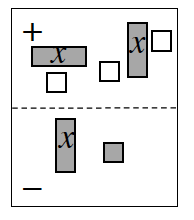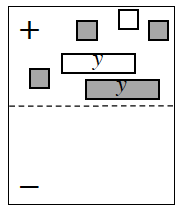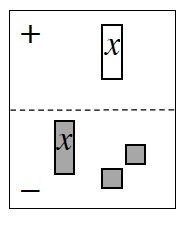### Home > INT1 > Chapter A > Lesson A.1.2 > ProblemA-20

A-20.

Write and simplify the algebraic expression shown in each Expression Mat below.1.$(2x − 3) − ( ? )$

Click the link at right for the full version of the eTool: Int1 A-20a HW eTool.

2.Which tiles pairs make zeroes?

1.$−x − (x + 2)$
$= −2x − 2$